Main Content

# Train TD3 Agent for PMSM Control

This example demonstrates speed control of a permanent magnet synchronous motor (PMSM) using a twin delayed deep deterministic policy gradient (TD3) agent.

The goal of this example is to show that you can use reinforcement learning as an alternative to linear controllers, such as PID controllers, in speed control of PMSM systems. Outside their regions of linearity, linear controllers often do not produce good tracking performance. In such cases, reinforcement learning provides a nonlinear control alternative.

Load the parameters for this example.

`sim_data`

Open the Simulink model.

```mdl = 'mcb_pmsm_foc_sim_RL'; open_system(mdl)```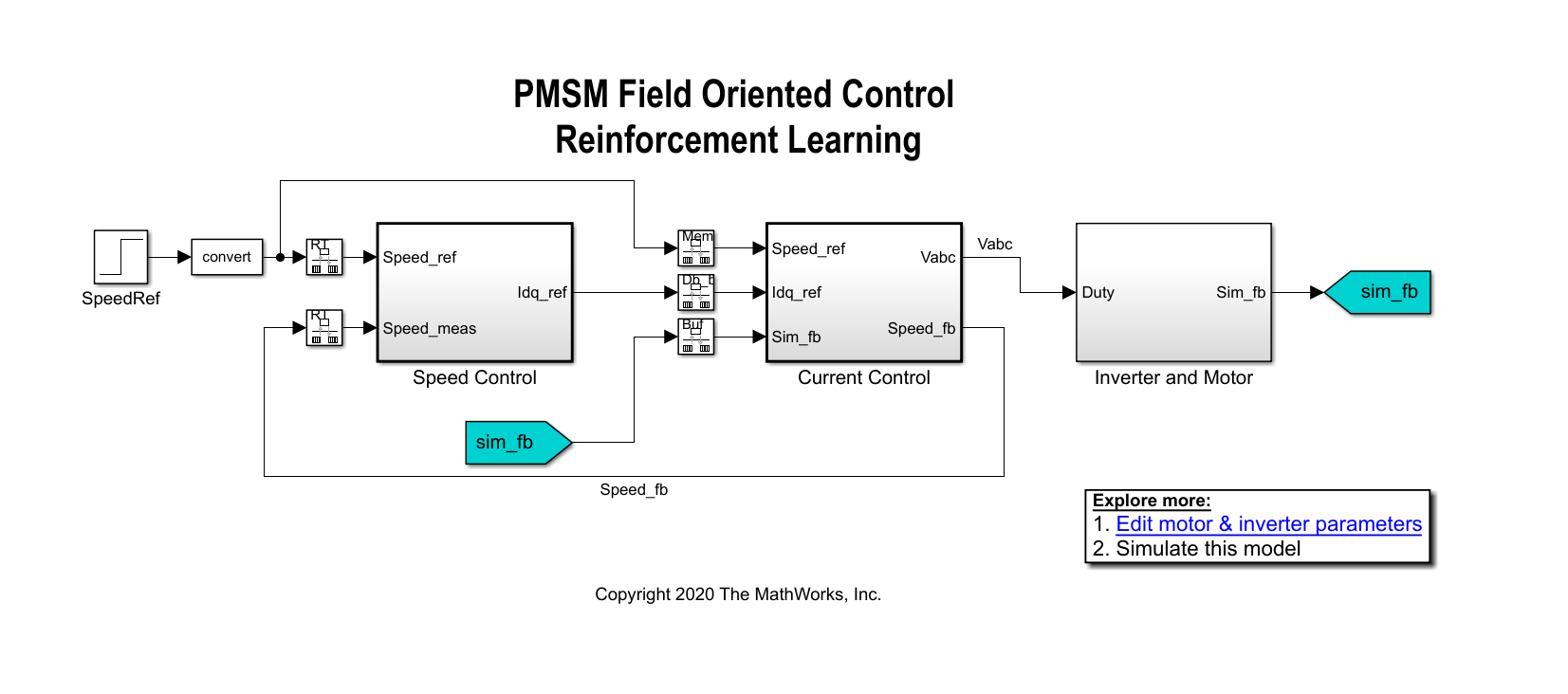In a linear control version of this example, you can use PI controllers in both the speed and current control loops. An outer-loop PI controller can control the speed while two inner-loop PI controllers control the d-axis and q-axis currents. The overall goal is to track the reference speed in the `Speed_Ref` signal. This example uses a reinforcement learning agent to control the currents in the inner control loop while a PI controller controls the outer loop.

### Create Environment Interface

The environment in this example consists of the PMSM system, excluding the inner-loop current controller, which is the reinforcement learning agent. To view the interface between the reinforcement learning agent and the environment, open the Closed Loop Control subsystem.

`open_system('mcb_pmsm_foc_sim_RL/Current Control/Control_System/Closed Loop Control')`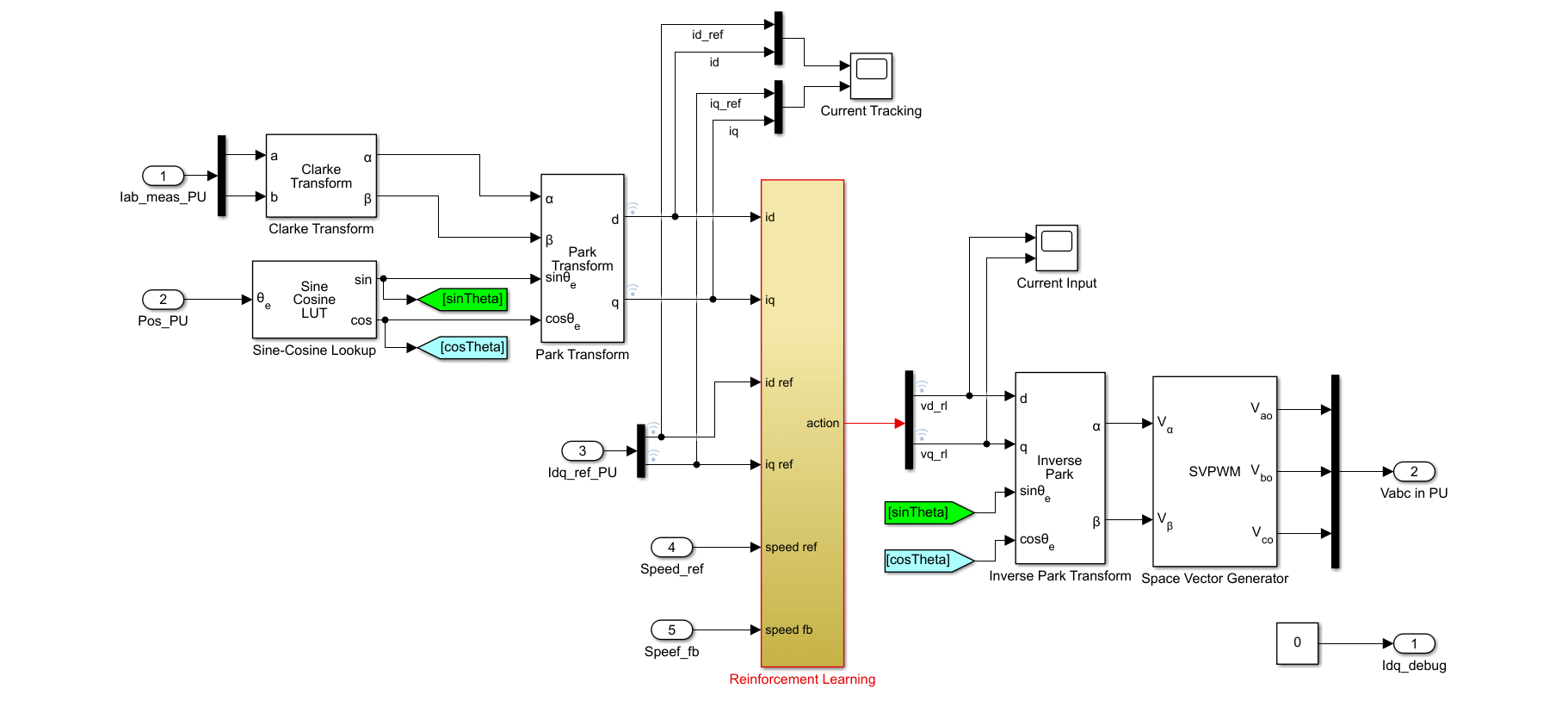The Reinforcement Learning subsystem contains an RL Agent block, the creation of the observation vector, and the reward calculation.

For this environment:

• The observations are the outer-loop reference speed `Speed_ref`, speed feedback `Speed_fb`, d-axis and q-axis currents and errors ($\mathrm{id}$, $\mathrm{iq}$, ${\mathrm{id}}_{\mathrm{error}}$ and ${\mathrm{iq}}_{\mathrm{error}}$), and the error integrals.

• The actions from the agent are the voltages `vd_rl` and `vq_rl`.

• The sample time of the agent is 2e-4 seconds. The inner-loop control occurs at a different sample time than the outer loop control.

• The simulation runs for 5000 time steps unless it is terminated early when the ${\mathrm{iq}}_{\mathrm{ref}}$ signal is saturated at 1.

• The reward at each time step is:

`${\mathit{r}}_{\mathit{t}}=-\left({\mathit{Q}}_{1}*{{\mathrm{id}}_{\mathrm{error}}}^{2}+{\mathit{Q}}_{2}*{{\mathrm{iq}}_{\mathrm{error}}}^{2}+\mathit{R}*\sum _{\mathit{j}}{{{\mathit{u}}^{\mathit{j}}}_{\mathit{t}-1}}^{2}\right)-100\mathit{d}$`

Here, ${\mathit{Q}}_{1}={\mathit{Q}}_{2}=5$, and $\mathit{R}=0.1$ are constants, ${\mathrm{id}}_{\mathrm{error}}$ is the d-axis current error, ${\mathrm{iq}}_{\mathrm{error}}$ is the q-axis current error, ${{\mathit{u}}^{\mathit{j}}}_{\mathit{t}-1}$ are the actions from the previous time step, and $\mathit{d}$ is a flag that is equal to 1 when the simulation is terminated early.

Create the observation and action specifications for the environment. For information on creating continuous specifications, see `rlNumericSpec`.

```% Create observation specifications. numObservations = 8; observationInfo = rlNumericSpec([numObservations 1]); observationInfo.Name = 'observations'; observationInfo.Description = 'Information on error and reference signal'; % Create action specifications. numActions = 2; actionInfo = rlNumericSpec([numActions 1]); actionInfo.Name = 'vqdRef';```

Create the Simulink environment interface using the observation and action specifications. For more information on creating Simulink environments, see `rlSimulinkEnv`.

```agentblk = 'mcb_pmsm_foc_sim_RL/Current Control/Control_System/Closed Loop Control/Reinforcement Learning/RL Agent'; env = rlSimulinkEnv(mdl,agentblk,observationInfo,actionInfo);```

Provide a reset function for this environment using the `ResetFcn` parameter. At the beginning of each training episode, the `resetPMSM` function randomly initializes the final value of the reference speed in the `SpeedRef` block to 695.4 rpm (0.2 pu), 1390.8 rpm (0.4 pu), 2086.2 rpm (0.6 pu), or 2781.6 rpm (0.8 pu).

`env.ResetFcn = @resetPMSM;`

### Create Agent

The agent used in this example is a twin-delayed deep deterministic policy gradient (TD3) agent. A TD3 agent approximates the long-term reward given the observations and actions using two critics. For more information on TD3 agents, see Twin-Delayed Deep Deterministic Policy Gradient Agents.

To create the critics, first create a deep neural network with two inputs (the observation and action) and one output. For more information on creating a value function representation, see Create Policy and Value Function Representations.

```rng(0) % fix the random seed statePath = [featureInputLayer(numObservations,'Normalization','none','Name','State') fullyConnectedLayer(64,'Name','fc1')]; actionPath = [featureInputLayer(numActions, 'Normalization', 'none', 'Name','Action') fullyConnectedLayer(64, 'Name','fc2')]; commonPath = [additionLayer(2,'Name','add') reluLayer('Name','relu2') fullyConnectedLayer(32, 'Name','fc3') reluLayer('Name','relu3') fullyConnectedLayer(16, 'Name','fc4') fullyConnectedLayer(1, 'Name','CriticOutput')]; criticNetwork = layerGraph(); criticNetwork = addLayers(criticNetwork,statePath); criticNetwork = addLayers(criticNetwork,actionPath); criticNetwork = addLayers(criticNetwork,commonPath); criticNetwork = connectLayers(criticNetwork,'fc1','add/in1'); criticNetwork = connectLayers(criticNetwork,'fc2','add/in2');```

Create the critic representation using the specified neural network and options. You must also specify the action and observation specification for the critic. For more information, see `rlQValueRepresentation`.

```criticOptions = rlRepresentationOptions('LearnRate',1e-4,'GradientThreshold',1); critic1 = rlQValueRepresentation(criticNetwork,observationInfo,actionInfo,... 'Observation',{'State'},'Action',{'Action'},criticOptions); critic2 = rlQValueRepresentation(criticNetwork,observationInfo,actionInfo,... 'Observation',{'State'},'Action',{'Action'},criticOptions);```

A TD3 agent decides which action to take given the observations using an actor representation. To create the actor, first create a deep neural network and construct the actor in a similar manner to the critic. For more information, see `rlDeterministicActorRepresentation`.

```actorNetwork = [featureInputLayer(numObservations,'Normalization','none','Name','State') fullyConnectedLayer(64, 'Name','actorFC1') reluLayer('Name','relu1') fullyConnectedLayer(32, 'Name','actorFC2') reluLayer('Name','relu2') fullyConnectedLayer(numActions,'Name','Action') tanhLayer('Name','tanh1')]; actorOptions = rlRepresentationOptions('LearnRate',1e-3,'GradientThreshold',1,'L2RegularizationFactor',0.001); actor = rlDeterministicActorRepresentation(actorNetwork,observationInfo,actionInfo,... 'Observation',{'State'},'Action',{'tanh1'},actorOptions);```

To create the TD3 agent, first specify the agent options using an `rlTD3AgentOptions` object. The agent trains from an experience buffer of maximum capacity 2e6 by randomly selecting mini-batches of size 512. Use a discount factor of 0.995 to favor long-term rewards. TD3 agents maintain time-delayed copies of the actor and critics known as the target actor and critics. Configure the targets to update every 10 agent steps during training with a smoothing factor of 0.005.

```Ts_agent = Ts; agentOptions = rlTD3AgentOptions("SampleTime",Ts_agent, ... "DiscountFactor", 0.995, ... "ExperienceBufferLength",2e6, ... "MiniBatchSize",512, ... "NumStepsToLookAhead",1, ... "TargetSmoothFactor",0.005, ... "TargetUpdateFrequency",10);```

During training, the agent explores the action space using a Gaussian action noise model. Set the noise variance and decay rate using the `ExplorationModel` property. The noise variance decays at the rate of 2e-4, which favors exploration towards the beginning of training and exploitation in later stages. For more information on the noise model, see `rlTD3AgentOptions`.

```agentOptions.ExplorationModel.Variance = 0.05; agentOptions.ExplorationModel.VarianceDecayRate = 2e-4; agentOptions.ExplorationModel.VarianceMin = 0.001;```

The agent also uses a Gaussian action noise model for smoothing the target policy updates. Specify the variance and decay rate for this model using the `TargetPolicySmoothModel` property.

```agentOptions.TargetPolicySmoothModel.Variance = 0.1; agentOptions.TargetPolicySmoothModel.VarianceDecayRate = 1e-4;```

Create the agent using the specified actor, critics, and options.

`agent = rlTD3Agent(actor,[critic1,critic2],agentOptions);`

### Train Agent

To train the agent, first specify the training options using `rlTrainingOptions`. For this example, use the following options.

• Run each training for at most 1000 episodes, with each episode lasting at most `ceil(T/Ts_agent)` time steps.

• Stop training when the agent receives an average cumulative reward greater than -190 over 100 consecutive episodes. At this point, the agent can track the reference speeds.

```T = 1.0; maxepisodes = 1000; maxsteps = ceil(T/Ts_agent); trainingOpts = rlTrainingOptions(... 'MaxEpisodes',maxepisodes, ... 'MaxStepsPerEpisode',maxsteps, ... 'StopTrainingCriteria','AverageReward',... 'StopTrainingValue',-190,... 'ScoreAveragingWindowLength',100);```

Train the agent using the `train` function. Training this agent is a computationally intensive process that takes several minutes to complete. To save time while running this example, load a pretrained agent by setting `doTraining` to `false`. To train the agent yourself, set `doTraining` to `true`.

```doTraining = false; if doTraining trainingStats = train(agent,env,trainingOpts); else load('rlPMSMAgent.mat') end```

A snapshot of the training progress is shown in the following figure. You can expect different results due to randomness in the training process.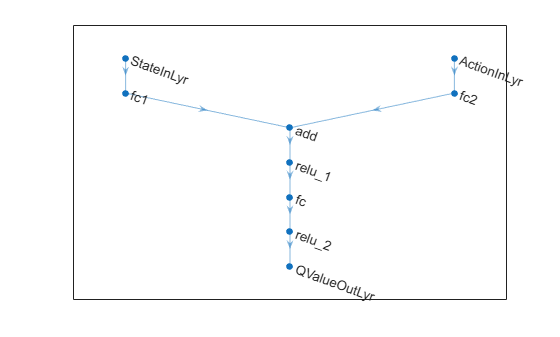### Simulate Agent

To validate the performance of the trained agent, simulate the model and view the closed-loop performance through the Speed Tracking Scope block.

`sim(mdl);`

You can also simulate the model at different reference speeds. Set the reference speed in the SpeedRef block to a different value between 0.2 and 1.0 per-unit and simulate the model again.

```set_param('mcb_pmsm_foc_sim_RL/SpeedRef','After','0.6') sim(mdl);```

The following figure shows an example of closed-loop tracking performance. In this simulation, the reference speed steps through values of 695.4 rpm (0.2 per-unit) and 1738.5 rpm (0.5 pu). The PI and reinforcement learning controllers track the reference signal changes within 0.5 seconds.

Although the agent was trained to track the reference speed of 0.2 per-unit and not 0.5 per-unit, it was able to generalize well.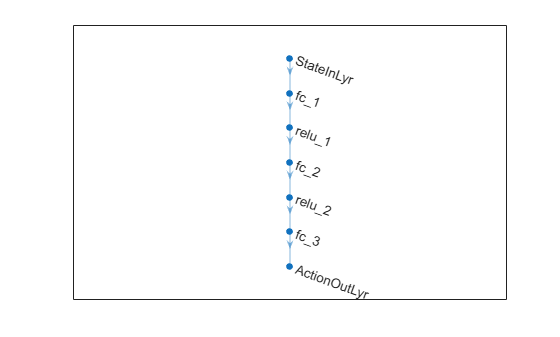The following figure shows the corresponding current tracking performance. The agent was able to track the $\mathrm{id}$ and $\mathrm{iq}$ current references with steady-state error less than 2%.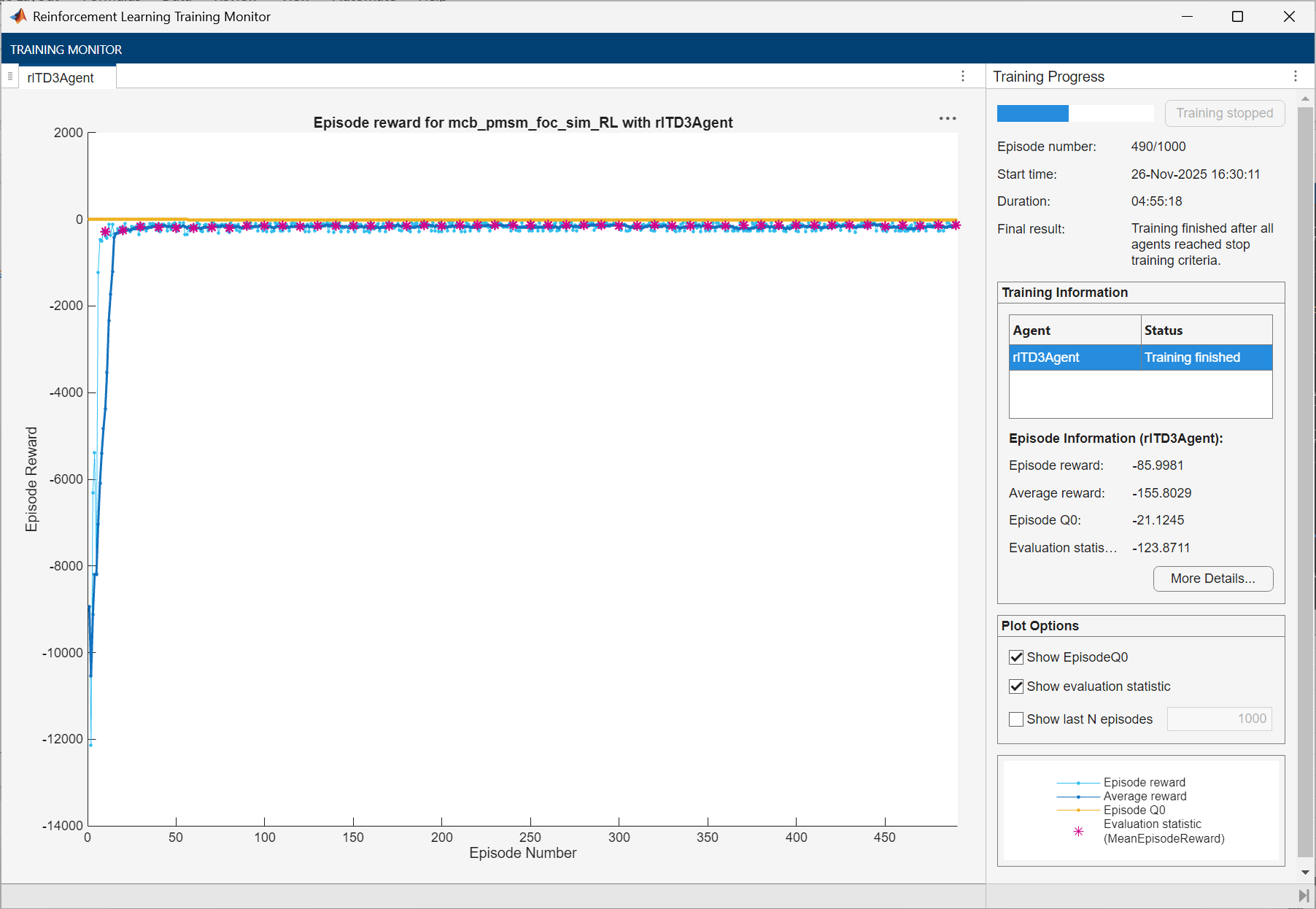Download ebook